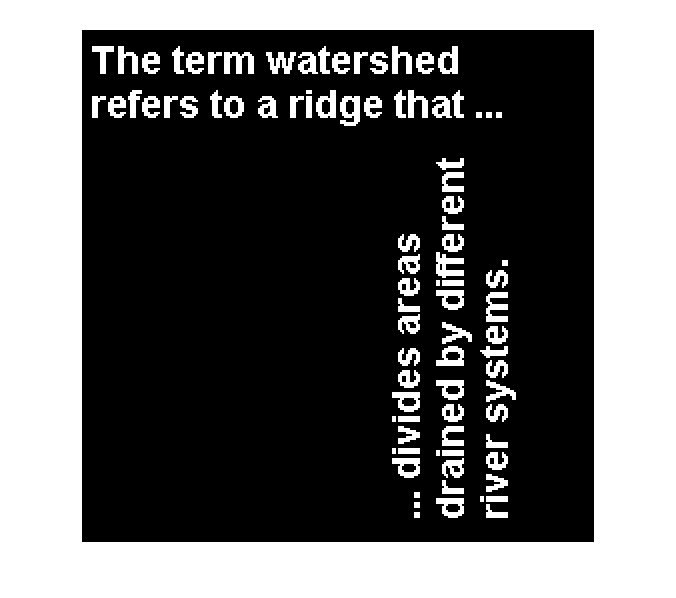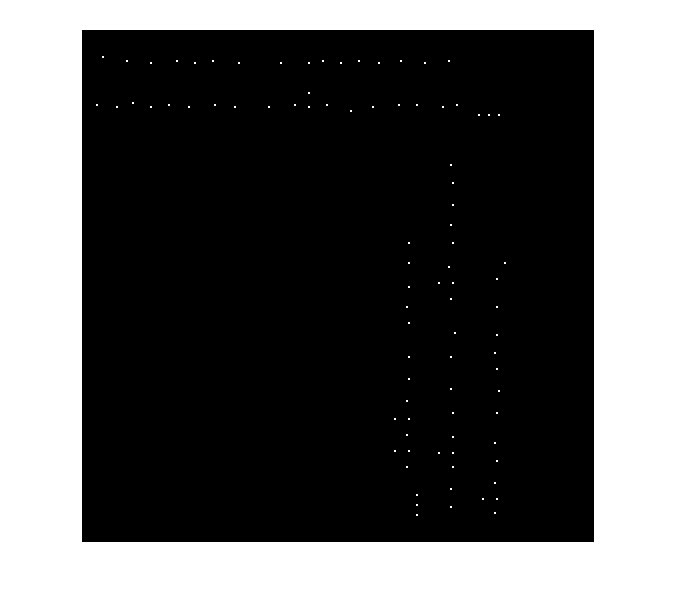# Modifying centroid locations in an image – an application of linear indexing

Blog reader Mike posed the following question recently:

If you have a bunch of point locations (for example, object centroids), how you make a binary image containing just those points?

For example, consider this image:

bw = imread('text.png');
imshow(bw, 'InitialMagnification', 200)How can we make an image like this, where the dots are located at the centroids of the objects?Solving this problem is a nice application of linear indexing, something I wrote about in this blog a long time ago. Let's see how it can work for us here.

First, let's find the centroids using regionprops:

s = regionprops(bw, 'Centroid');

s is a struct array. Since we just asked for one measurement, the centroid, each element of s is a struct containing just one field, 'Centroid'.

s(1)
ans =
Centroid: [11 13.5000]

s(2)
ans =
Centroid: [7.6829 38.1707]


The length of s is the number of objects in the image.

num_objects = length(s)
num_objects =
88


Next, we gather all the individual centroid locations into x and y vectors. To accomplish this I use the comma-separated list syntax for struct arrays.

centroids = cat(1, s.Centroid);
x = centroids(:,1);
y = centroids(:,2);

If the comma-separated list syntax makes your brain hurt, you can use a loop instead:

  centroids = zeros(length(s), 2);
for k = 1:length(s)
centroids(k,:) = s(k).Centroid;
end

Now let's round the centroid locations to get row and column subscripts.

r = round(y);
c = round(x);

Here's where linear indexing comes into play. In order to assign to a bunch of scattered locations like this, you want to use a single subscript. That's what we call linear indexing. You can use the function sub2ind to convert a set of subscripts to linear indices.

ind = sub2ind(size(bw), r, c);

And finally we can use the linear indices to assign a value to a bunch of image pixel locations all at once.

bw2 = false(size(bw));
bw2(ind) = true;

imshow(bw2, 'InitialMagnification', 200)See my 08-Feb-2008 blog post for more about linear indexing.

Published with MATLAB® 7.13

|